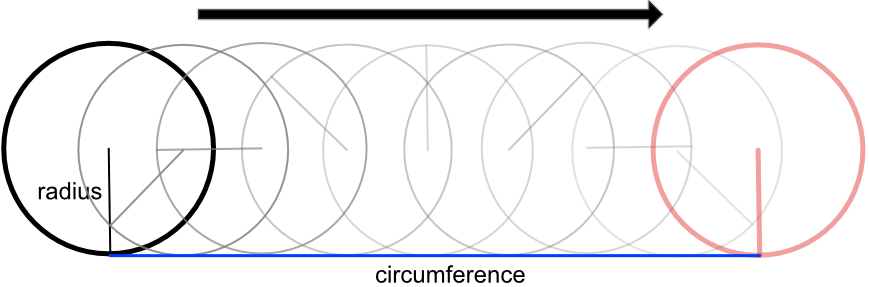Circumference of a Circle

## Circumference of a Circle

When the radius of a circle is known, the circumference can be calculated by using a formula.

The formula is C = 2pir, where C is the circumference and r is the radius.

The value of π = 3.14159 (to 5dp).

If a diameter is given use C = pid. This is the same formula, as 2r = d.

A circumference is a linear measurement; that is, it is a line. The units for a circumference will be, for example, in cm, metres or inches.## Example 1

What is the circumference of this circle? Give your answer to 1 decimal place.Circumference of a circle = 2pir

C = 2 x 3.14 x 12 = 75.36, or 75.4 to 1dp

## Example 2

A mark is made on a wheel. The wheel is placed such that the mark touches the ground. The wheel is then rolled until the mark touches the ground again. The distance between the two marks is 200cm. What is the radius of the wheel? Give your answer to 2 decimal places.

The wheel rolling along the ground describes the circumference as it rolls. The distance between the two marks is therefore the circumference.

C = 2pir

200 = 2 x 3.14 x r

r = 31.847, or 31.85 to 2dp